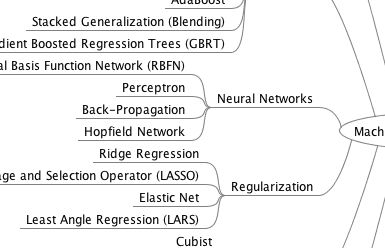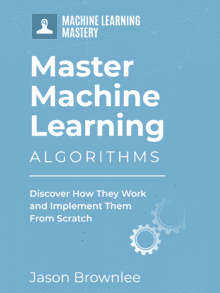# How to Study Machine Learning Algorithms

Algorithms make up a big part of machine learning.

You select and apply machine learning algorithms to build a model from your data, select features, combine the predictions from multiple models and even evaluate the capabilities of a given model.

In this post you will review 5 different approaches that you can use to study machine learning algorithms.

Kick-start your project with my new book Master Machine Learning Algorithms, including step-by-step tutorials and the Excel Spreadsheet files for all examples.

## 1. List Machine Learning Algorithms

There are a lot of machine learning algorithms and it can feel overwhelming.

Even defining what a machine learning algorithm is, can be tricky.

A great place to start out is to make your own lists of algorithms. Start a text file, word document or spreadsheet and list algorithm names. Also list the general category or categories to which each algorithm belongs.

This simple tactic can help you build familiarity with the different types and classes of algorithms available. Later as you get more experienced, lists like this can prompt you and give you ideas of different methods to spot check on your problem.

Some examples of algorithm lists to get you started include:

## Get your FREE Algorithms Mind MapSample of the handy machine learning algorithms mind map.

I've created a handy mind map of 60+ algorithms organized by type.

## 2. Apply Machine Learning Algorithms

Machine Learning algorithm do not exist in isolation, they are best understood when applied to a dataset.

Apply algorithms to problems to understand them. Practice applied machine learning. It sounds simple, but you will be amazed at the number of people paralyzed to make this small step from theory into action.

This may mean working on a problem that matters to you, a competition dataset or a classical machine learning dataset.

Use a machine learning platform like Weka, R or scikit-learn to get access to many machine learning algorithms.

Start to build up an intuition for different types of algorithms, such as decision trees and support vector machines. Think about their required preconditions and the effects the parameters have on results.

Build up confidence in applying different algorithms. You should be spot checking algorithms on your problems.

## 3. Describe Machine Learning Algorithms

The next step in understanding a machine learning algorithm is to explore what is already understood about the algorithm.

This could be done before you apply the algorithm, but I think it is valuable to have a working intuition of the algorithm in action as context before diving into the algorithm description.

You can research an algorithm. This includes locating and reading the primary sources where the algorithm was first described as well as authoritative interpretations of the algorithm in textbooks and review papers.

Conference papers, competition results and even forms and Q&A websites can help you better understand the best practices and usage heuristics for an algorithm.

As you are researching an algorithm, build up a description. I like to use a well-defined algorithm description template.

You can continue to add to this template you discover more about an algorithm. You can add references, list the pseudocode for the algorithm and list best practices and usage heuristics.

This is a valuable techniques and you can build up your own mini-encyclopedia of algorithm descriptions for your own reference (for example, see Clever Algorithms for 45 algorithm recipes).

For more information on the template that I use, check out the post “How to Learn a Machine Learning Algorithm“.

## 4. Implement Machine Learning Algorithms

Implementing machine learning algorithms is an excellent way to get a concrete understanding of how an algorithm works.

There are many micro-decisions that have to be made when implementing an algorithm. Some of these decision points are exposed with algorithm configuration parameters, but many are not.

By implementing an algorithm yourself you will get a feeling for just how to customize the algorithm and choose what to expose and what decision points to fix in place.

Implementing algorithms from scratch will help you understand the mathematical descriptions and extensions of an algorithm. This may sound counter-intuitive. The mathematical descriptions are idealized and often provide a snap-shot description of a given processes within an algorithm. Once you translate them into code, the implications of those descriptions may be a lot more obvious.

You can leverage tutorials and open source implementations of algorithms to help you get through those difficult parts.

Note that a “my first implementation” of an algorithm will be less scalable and more fragile than a production grade implementation you may find in a machine learning tool or library.

Take a look at my tutorial for implementing k-nearest neighbors from scratch in Python.

You may also be interested in my post “How to Implement a Machine Learning Algorithm“.

## 5. Experiment On Machine Learning Algorithms

Experimenting on machine learning algorithms is the best way to understand them.

You need to act like the scientist and study a running machine learning algorithm like a complex system.

You need to control variables, use standardized datasets that are well understood and explore the cause and effect relationships of the parameters on the results.

Understanding the effects of the parameters will help you better configure the algorithm on problems in the future. Understanding the behavior of the algorithm under different circumstances will help you better scale and adapt the method to new and different problem domains in the future.

Many machine learning algorithms are stochastic in nature and resist more classical methods of algorithm analysis. They often require empirical investigation and probabilistic description to be understood.

## Summary

In this post you discovered five ways to study and learn about machine learning algorithms.

They where:

1. List Machine Learning Algorithms
2. Apply Machine Learning Algorithms
3. Describe Machine Learning Algorithms
4. Implement Machine Learning Algorithms
5. Experiment On Machine Learning Algorithms

Have you used any of these methods to learn a machine learning algorithm?

Perhaps you have your own approach to studying machine learning algorithms? Share it in the comments below.

## Discover How Machine Learning Algorithms Work!#### See How Algorithms Work in Minutes

...with just arithmetic and simple examples

Discover how in my new Ebook:
Master Machine Learning Algorithms

It covers explanations and examples of 10 top algorithms, like:
Linear Regression, k-Nearest Neighbors, Support Vector Machines and much more...

#### Finally, Pull Back the Curtain on Machine Learning Algorithms

1.